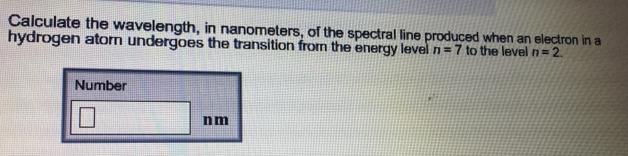# Problem: Calculate the wavelength, in nanometers, of the spectral line produced when an electron in a hydrogen atom undergoes the transition from the energy level n = 7 to the level n = 2.

###### FREE Expert Solution
80% (454 ratings)###### Problem Details

Calculate the wavelength, in nanometers, of the spectral line produced when an electron in a hydrogen atom undergoes the transition from the energy level n = 7 to the level n = 2.